# p-values with large samples (AMA)

Vishnu K, a doctoral student in Finance writes in a question:

Dear Professor Andrew Wheeler

Hope you are fine. I am big follower of your blog and have used it heavily to train myself. Since you welcome open questions, I thought of asking one here and I hope you don’t mind.

I was reading the blog Dave Giles and one of his blogs https://davegiles.blogspot.com/2019/10/everythings-significant-when-you-have.html assert that one must adjust for p values when working with large samples. In a related but old post, he says the same

“So, if the sample is very large and the p-values associated with the estimated coefficients in a regression model are of the order of, say, 0.10 or even 0.05, then this really bad news. Much, much, smaller p-values are needed before we get all excited about ‘statistically significant’ results when the sample size is in the thousands, or even bigger. So, the p-values reported above are mostly pretty marginal, as far as significance is concerned” https://davegiles.blogspot.com/2011/04/drawing-inferences-from-very-large-data.html#more

In one of the posts of Andrew Gelman, he said the same

“When the sample size is small, it’s very difficult to get a rejection (that is, a p-value below 0.05), whereas when sample size is huge, just about anything will bag you a rejection. With large n, a smaller signal can be found amid the noise. In general: small n, unlikely to get small p-values.

Large n, likely to find something. Huge n, almost certain to find lots of small p-values” https://statmodeling.stat.columbia.edu/2009/06/18/the_sample_size/

As Leamer (1978) points if the level of significance should be set as a decreasing function of sample size, is there a formula through which we can check the needed level of significance for rejecting a null?

Context 1: Sample Size is 30, number of explanatory variables are 5

Context 2: Sample Size is 1000, number of explanatory variables are 5

In both contexts cant, we use p-value <.05 or should we fix a very small p-value for context 2 even though both contexts relates to same data set with difference in context 2 being a lot more data points!

Worrying about p-values here is in my opinion the wrong way to think about it. You can focus on the effect size, and even if an effect is significant, it may be substantively too small to influence how you use that information.

Finance I see, so I will try to make a relevant example. Lets say a large university randomizes students to take a financial literacy course, and then 10 years later follows up to see their overall retirement savings accumulated. Say the sample is very large, and we have results of:

``````Taken Class: N=100,000 Mean=5,000 SD=2,000
No Class: N=100,000 Mean=4,980 SD=2,000

SE of Difference ~= 9
Mean Difference = 20
T-Stat ~= 2.24
p-value ~= 0.025``````

So we can see that the treated class saves more! But it is only 20 dollars more over ten years. We have quite a precise estimate. Even though those who took the class save more, do you really think taking the class is worth it? Probably not based on these stats, it is such a trivial effect size given the sample and overall variance of savings.

And then as a follow up from Vishnu:

Thanks a lot Prof Andrew. One final question is, Can we use the Cohen’s d or any other stats for effect size estimation in these cases?

Cohen’s d = (4980 – 5000) ⁄ 2000 = 0.01.

I don’t personally really worry about Cohen’s D to be honest. I like to try to work out the cost-benefits on the scales that are meaningful (although this makes it difficult to compare across different studies). So since I am a criminologist, I will give a crime example:

``````Treated Areas: 40 crimes
Non-Treated Areas: 50 crimes``````

Ignore the standard error for this at the moment. Whether a drop in 10 crimes “is worth it” depends on the nature of the treatment and the type of crime. If the drop is simply stealing small items from the store, but the intervention was hire 10 security guards, it is likely not worth it (the 10 guards salary is likely much higher than the 10 items they prevented theft of).

But pretend now that the intervention was nudging police officers to patrol more in hot spots (so no marginal labor cost) and the crimes we examined were shootings. Preventing 10 shootings is a pretty big deal, because they have such large societal costs.

In this scenario the costs-benefits are always on the count scale (how many crimes did you prevent). Doing another scale (like Cohen’s D or incident rate ratios or whatever) just obfuscates how to calculate the costs/benefits in this scenario.

# Potential IT student projects with police? (AMA)

Abigail White writes in:

I’m still settling in at my agency and getting an analysis program rolling. Before I came here, I volunteered with a nonprofit and I had partnered 2 years running with a local university to provide real-world opportunities for their 4th-year undergrad IT students to do a final project. Basically, I was the project owner and they were supposed to build an application, modify software for my needs, or do some project along those lines to get on-the-ground experience. The school just reached out again and asked if I wanted to do a project with them again this year with whatever organization I’m currently working with.

I really want to tell them yes because it’s a tremendous opportunity for these students to know about how IT can interface with the field of law enforcement (and to get some IT minds working on law enforcement problems). Plus my agency actually may want to hire in-house IT at some point so it could be a good way for them to screen some people and make a job offer at the end of the school year.

Do you have any ideas for projects that would be good to build bridges between the IT industry and law enforcement? I need to come up with something good before I pitch it to command. The best I can think of so far is a phone-based app where officers could record the lat and long of their exact location and add info about the environment around them – ultimately so we could start getting good data for risk terrain modeling (i.e., at this location, there’s an abandoned house, at another location, there’s an unoccupied house where the lawn hasn’t been mown, etc.) I know that’s not much to go on, but I would love to hear any thoughts you have!

And here is what I said off-the-cuff in response:

It is hard for me to gauge feasibility. In terms of app maybe a nice public facing dashboard (maps/charts), with public info. Can pitch as something for community group meetings for example. That is a good marriage between IT (worrying about the server) and crime analysis (queries to feed the dashboard) I think.

Phone app to me sounds too hard. Even if you build it very difficult to get data security down and the PD to actually use it. Public dashboard is for everyone and no security worries. (Building a phone app seems pretty far outside what an IT person would typically do within a PD.)

RTM in terms of building a predictive model is feasible, but making a nice way for the PD to consume it is more difficult (so is more traditional crime analysis type stuff). But could maybe make sense just internally.

I have seen someone try to do the video narratives with officers (similar to your app idea, just using gopro). It is neat, but not sure it results in very actionable intelligence to be honest.

This is just me rambling though. Typically for projects I start with something the PD will find useful based on my own observations. So would look for regular things done low-tech now by the PD that can maybe be automated or improved upon with some coding work.

Sharing here with the hopes others will have more ideas. It is similar in scope to say a really good undergrad or a masters level thesis type project I would say as well.

# Solving the P-Median model

Wendy Jang, my former student at UTD and now Data Scientist for the Stanislaus County Sheriff’s Dept. writes in:

Hello Andy,

I have some questions about your posting in GitHub. Honestly, I am not good at Python at all. I was playing with your python code and trying to understand the work flow. Then, I can pretty much mimic what you did; however, I encountered some errors along the way.

I was able to follow through lines but then I am stuck on PMed function. Do you know what possibly caused this error? See the screenshot below.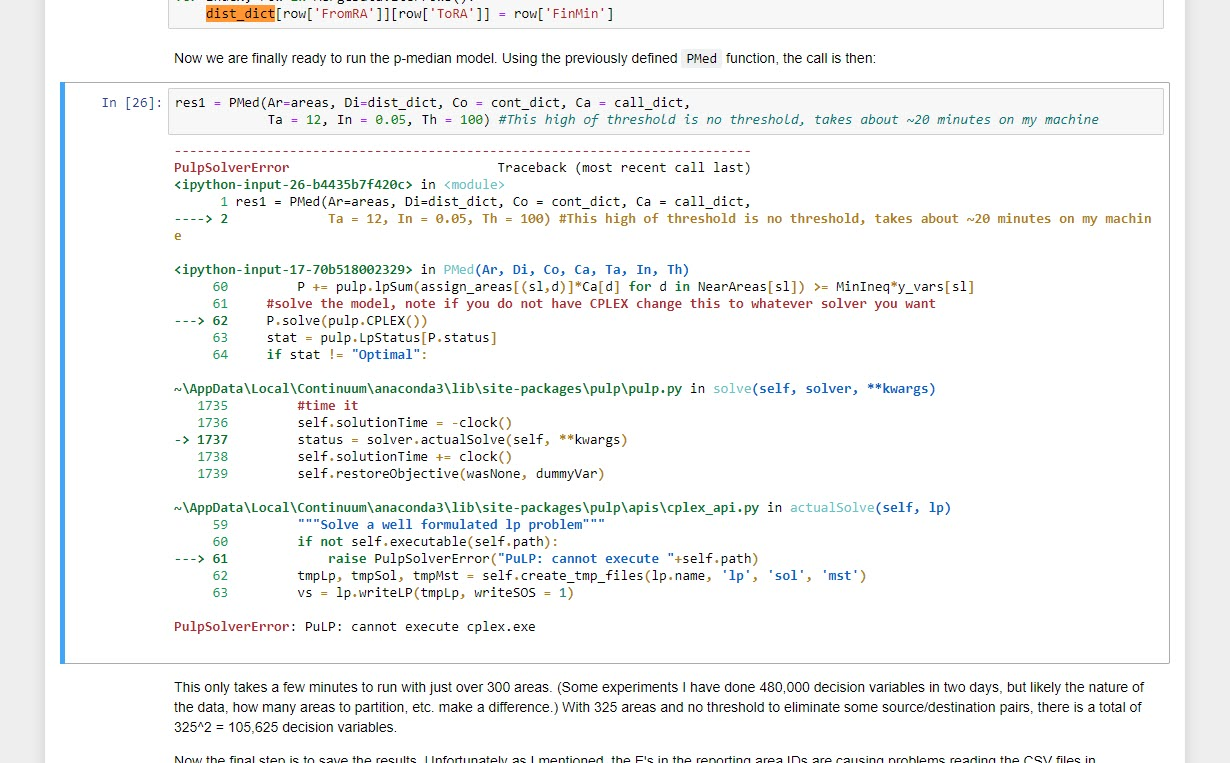Please advise. Thanks!

Specifically, Wendy is asking about my patrol redistricting example with workload inequality constraints. Wendy’s problem here is specifically she likely does not have CPLEX installed. CPLEX is free for academics, but unfortunately costs a bit of money for anyone else (not sure if they have cheaper licensing for public sector, looks like currently about \$200 a month).

This was a good opportunity to update some of my code, so now see the two `.py` files in the DataCreated folder, in particular `01_pmed_class.py` has a nice class function. I have additionally added in functionality to create a map, and more importantly eliminate subtours (my solution can potentially return disconnected areas). I have also added the ability to use different solvers.

For this problem CPLEX just works much better (takes around 10 minutes), but I was able to get the SCIP solver to return the same solution in around 5 hours. GLPK did not return a solution even letting it churn out for over 12 hours. I tested CBC for shorter time periods, but that appears to be a no-go as well.

So just a brief overview, the libraries I will be using (check out the end of the post for setting up the conda environment):

``````import pickle
import pulp
import networkx
import pandas as pd
from datetime import datetime
import matplotlib.pyplot as plt
import geopandas as gpd

class pmed():
"""
Ar - list of areas in model
Di - distance dictionary organized Di[a1][a2] gives the distance between areas a1 and a2
Co - contiguity dictionary organized Co[a1] gives all the contiguous neighbors of a1 in a list
Ca - dictionary of total number of calls, Ca[a1] gives calls in area a1
Ta - integer number of areas to create
In - float inequality constraint
Th - float distance threshold to make a decision variables
"""
def __init__(self,Ar,Di,Co,Ca,Ta,In,Th):``````

I do not copy-paste the entire `pmed` class in the blog post. It is long, but is the rewrite of my model from the paper. Next, to load in the objects I need to fit the model (which were created/pickled in the `00_PrepareData.py` file).

``````# Loading in data
data_loc = r'D:\Dropbox\Dropbox\PublicCode_Git\PatrolRedistrict\PatrolRedistrict\DataCreated\fin_obj.pkl'
areas, dist_dict, cont_dict, call_dict, nid_invmap, MergeData = pickle.load(open(data_loc,"rb"))

# shapefile for reporting areas

And now we can create a `pmed` object, which has all the info necessary, and gives us a print out of the total number of decision variables and constraints.

``````# Creating pmed object
pmed12 = pmed(Ar=areas,Di=dist_dict,Co=cont_dict,Ca=call_dict,Ta=12,In=0.1,Th=10)``````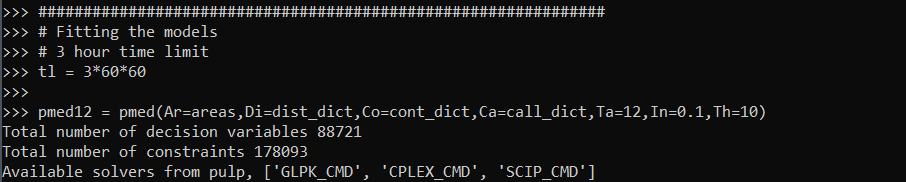Writing the class this way allows the user to input their own solver, and you can see it prints out the available solvers on your current machine. So to pass in the SCIOPT solver you could do `pmed12.solve(solver=pulp.SCIP_CMD())`, which again for this problem does find the solution, but takes 5 hours. So here I still stick with CPLEX to just illustrate the new classes functionality:

``pmed12.solve(solver=pulp.CPLEX(timeLimit=30*60,msg=True))     # takes around 10 minutes``

This prints out a ton of information at the command line that you do not get if you run from within Jupyter notebooks. So for debugging that is much more useful. `timeLimit` is in seconds, but does not include the presolve phase I believe, so some of these solvers can just get stuck on the presolve forever.

We can look at the results in a nice map if you do have geopandas installed and pass it a geopandas data frame and the key to match it on:

``pmed12.map_plot(carr_report, 'PDGrid')``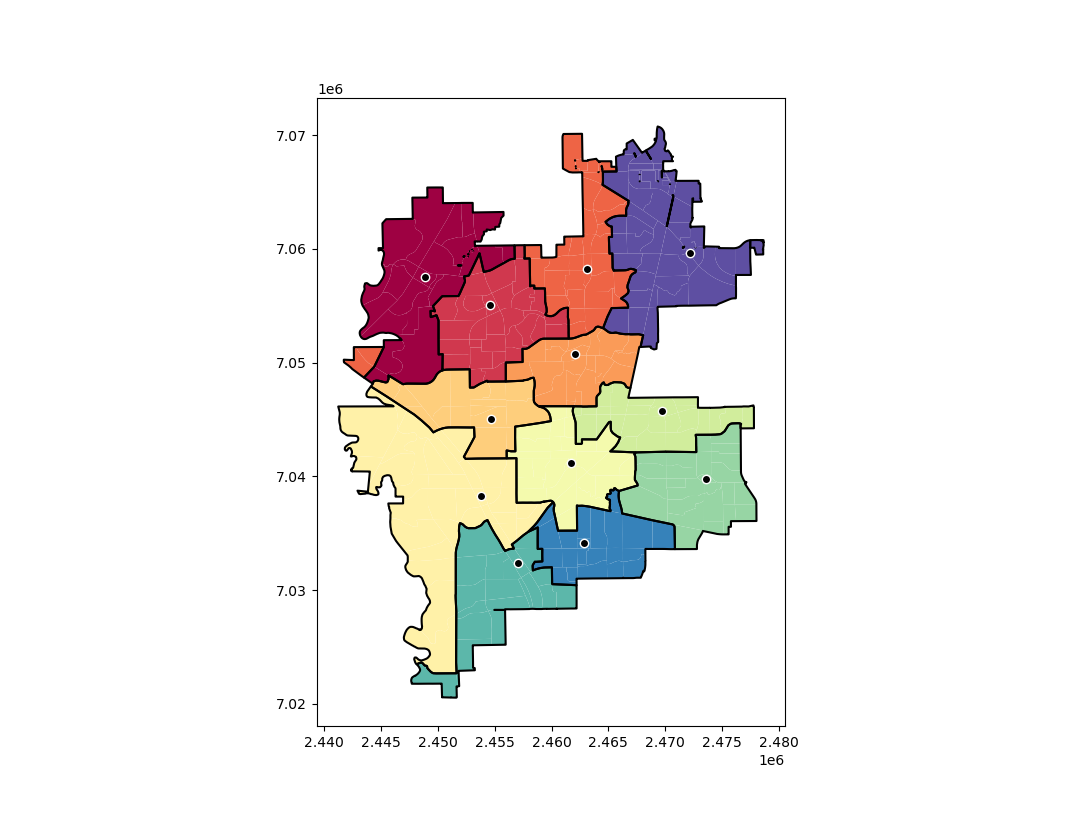This map shows the new areas and different colors with a thick border, and the source area as a dot with a white outline. So here you can see that we end up having one disconnected area – a subtour in optimization parlance. I have added some methods to deal with this though:

``stres = pmed12.collect_subtours()``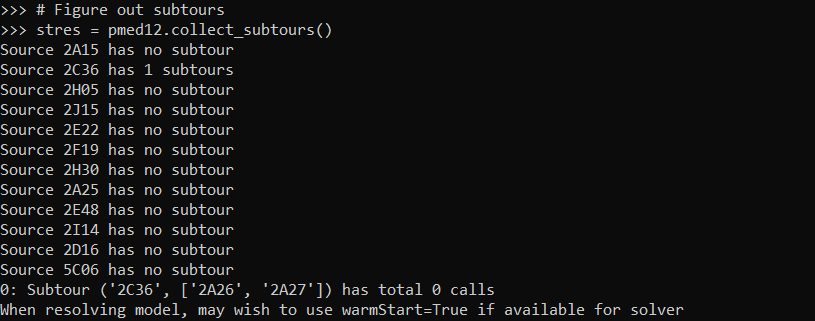And you can see that for one of our areas it identified a subtour. I haven’t written this to automatically resolve for subtours due to the potential length of the solving time. So here we can see the subtours have 0 calls in them. You can assign those areas to wherever and it does not change the objective function. So that is what I did in the paper and showed how in the Jupyter notebook at the main page for the github project.

So if you are using a function that takes 5 hours, I would suggest you manually fix these subtours if there is an obvious to the human eye easy solution. But here with the shorter solve time with CPLEX I can rerun the algorithm with a warm start, and it runs even faster (under 5 minutes). The `.collect_subtours()` method adds subtour constraints into the pulp model, so you just need to redo the `solve()` method to eliminate that particular subtour (I do not know if this is guaranteed to converge though for all problems).

So here in this data eliminating that one subtour results in a solution with no subtours:

``````pmed12.solve(solver=pulp.CPLEX_CMD(msg=False,warmStart=True))
stres = pmed12.collect_subtours()``````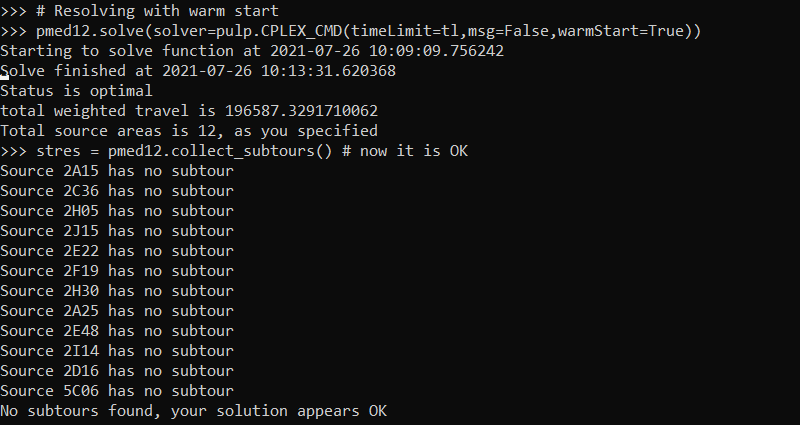And we can replot the result, which shows it chooses the areas that you would have assigned by eye anyway:

``pmed12.map_plot(carr_report, 'PDGrid')``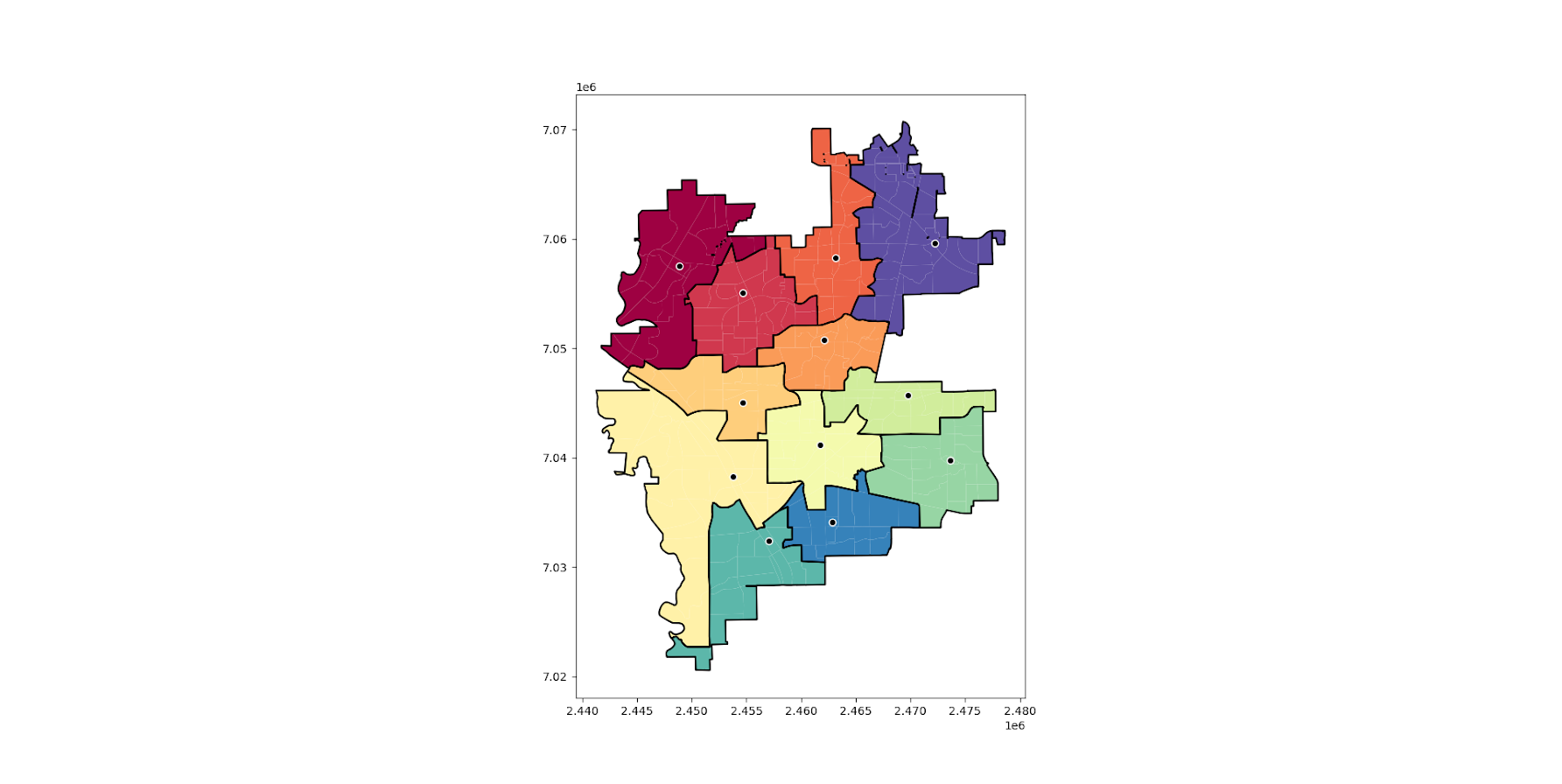So again for this problem if you have CPLEX I would recommend it (have not tried Gurobi). But at least for this particular dataset SCIOPT was able to solve the problem in 5 hours. So if you are a crime analyst or someone else without academic access to CPLEX you can install the sciopt solver and give that a go for your actual data.

Note that this is based off of results for 325 subareas. So if you have more subareas it will take a longer (and if you have fewer it may be quite a bit shorter).

# Setting up the Conda environment

So once you have python installed, you can typically do something like:

``pip install pulp``

Or via conda:

``conda install pyscipopt``

I often have trouble though, especially when working with the python geospatial libraries, to install geopandas, fiona, etc. So here what I do is create a new conda environment:

``````conda create -n linprog
conda activate linprog
conda install -c conda-forge python=3 pip pandas numpy networkx scikit-learn dbfread geopandas glpk pyscipopt pulp``````

And then you can run the pmedian code above in this environment. I suppose I should turn this into a python package, and I see a bunch of folks anymore are doing docker images as well with their packages in complicated environments. This is actually not that bad, minus geopandas makes things a bit tricky.

# Difference in independent effects for multivariate analysis (SPSS)

For some reason my various posts on testing differences in coefficients are fairly high in google search results. Arden Roeder writes in with another related question on this:

Good evening Dr. Wheeler,

I am a PhD candidate at the University of Oklahoma working on the final phases of data analysis for my dissertation. I found an article on your website that almost answers a question I have about a potential statistical test, and I’m hoping you might be able to help me fill in the gaps.

If you are willing to help me out, I would greatly appreciate it, and if not, I completely understand!

Here’s the setup: I have two independent variables (one measured on a 6-point Likert scale, the other on a 7-point) and six dependent variables. I have hypothesized that IV1 would be a stronger predictor of three of the DVs, and that IV2 would be a stronger predictor of the other three DVs. I ran multiple linear regression tests for each of the DVs, so I have the outputs for those. I know I can compare the standardized betas just to see strength, but what I’d like to know is how I can determine whether the difference between the beta weights is significant, and then to assess this for each of the six DVs.

From reading through your post, it seems like the fourth scenario you set up is quite close to what I’m trying to do, but I’m not sure how to translate the covariance output I have (I’m using SPSS) to what you’ve displayed here. Can I simply square the standard errors I have to get the numbers on the diagonal, and then grab the covariance from the SPSS output and solve accordingly? (I also reviewed your writing here about using the GLM procedure as well, but can’t seem to align my outputs with your examples there either.)

Here’s a sample of the numbers I’m working with: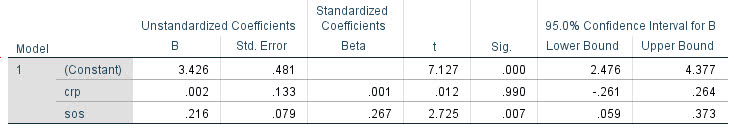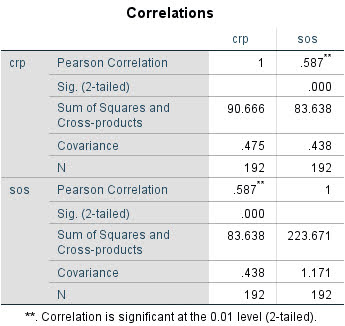Any insights you can offer on 1) whether this is the right test to give me the answers I’m looking for about whether the betas are significantly different and 2) how to set up and interpret the results correctly would be a tremendous help.

For 1, yes I think this is an appropriate way to set up the problem. For 2, if sticking to SPSS it is fairly simple syntax in GLM:

``````*****************************.
*Contrasts with X1 - X2 effect across the variables.
GLM Y1 Y2 Y3 Y4 Y5 Y6 WITH X1 X2
/DESIGN=X1 X2
/PRINT PARAMETER
/LMATRIX = "T1"
X1  1
X2 -1.
*****************************.``````

To get this to do the standardized coefficients, Z-score your variables before the GLM command (this is assuming you are estimating a linear model, and not a non-linear model like logit/Poisson). (I have full simulation SPSS code at the end of the post illustrating.)

Note that when I say the covariance between beta coefficients, this is NOT the same thing as the covariance between the original metrics. So the correlation matrix for X1 vs X2 here does not give us the information we need.

For 2, the reporting part, you can see the Contrast results K matrix table in SPSS. I would just transpose that table, make a footnote/title this is testing X1 – X2, and then just keep the columns you want. So here is the original SPSS contrast table for this result: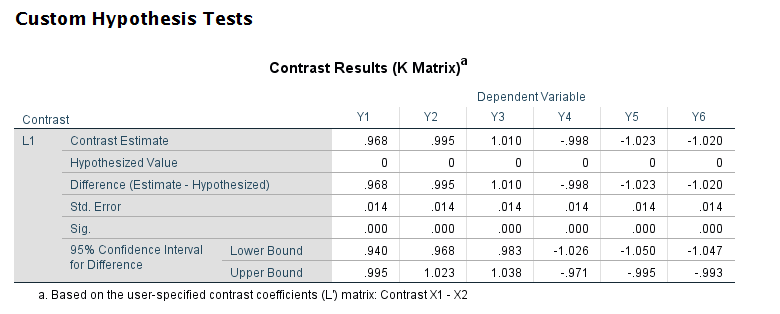And here is how I would clean up the table and report the tests: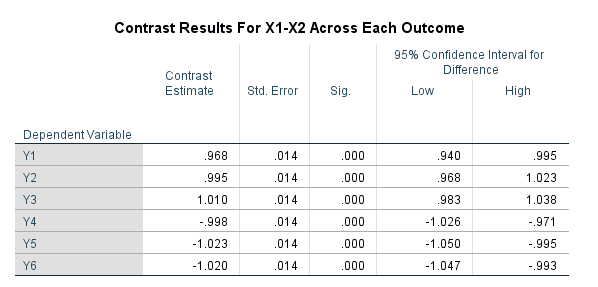# SPSS Simulation Code

Here I just simulate independent X’s, and give the Y’s consistent effects. You could also simulate multivariate data with specified correlations if you wanted to though.

``````****************************************************.
* Simulated data to illustrate coefficient diff.
* tests.
SET SEED 10.
INPUT PROGRAM.
LOOP #i = 1 TO 10000.
COMPUTE Id = #i.
END CASE.
END LOOP.
END FILE.
END INPUT PROGRAM.

COMPUTE X1 = RV.NORMAL(0,1).
COMPUTE X2 = RV.NORMAL(0,1).
COMPUTE Y1 = 1.5*X1 + 0.5*X2 + RV.NORMAL(0,1).
COMPUTE Y2 = 1.5*X1 + 0.5*X2 + RV.NORMAL(0,1).
COMPUTE Y3 = 1.5*X1 + 0.5*X2 + RV.NORMAL(0,1).
COMPUTE Y4 = 0.5*X1 + 1.5*X2 + RV.NORMAL(0,1).
COMPUTE Y5 = 0.5*X1 + 1.5*X2 + RV.NORMAL(0,1).
COMPUTE Y6 = 0.5*X1 + 1.5*X2 + RV.NORMAL(0,1).
EXECUTE.

*Contrasts with X1 - X2 effect across the variables.
GLM Y1 Y2 Y3 Y4 Y5 Y6 WITH X1 X2
/DESIGN=X1 X2
/PRINT PARAMETER
/LMATRIX = "Contrast X1 - X2"
X1  1
X2 -1.

*And here is an example stacking equations and using EMMEANS.
*Stacking equation approach, can work for various.
*Generalized linear models, etc.
VARSTOCASES
/MAKE Y FROM Y1 TO Y6
/Index Outcome.

GENLIN Y BY Outcome WITH X1 X2
/MODEL Outcome X1 X2 Outcome*X1 Outcome*X2
/CRITERIA COVB=ROBUST
/REPEATED SUBJECT=Id CORRTYPE=UNSTRUCTURED
/EMMEANS TABLES=Outcome CONTROL= X1(1) X2(-1).
****************************************************.``````

# How to interpret one sided tests for coefficient differences?

In my ask me anything series, Rob Case writes in a question about interpreting one-sided tests for the difference in coefficients:

Mr. Wheeler,

Thank you for your page https://andrewpwheeler.com/2016/10/19/testing-the-equality-of-two-regression-coefficients/

I did your technique (at the end of the page) of re-running the model with X+Z and X-Z as independent variables (with coefficients B1 and B2, respectively).

I understand:

1. (although you did not say so) that testing whether coefficient b1 (X’s coefficient in the original equation) is LESS THAN coefficient b2 (Z’s coefficient in the original regression) is a one-sided test; and testing whether one coefficient is DIFFERENT from another is a two-sided test
2. that the 90%-confidence t-distribution-critical-values-with-infinite-degrees-of-freedom are 1.282 for one-sided tests and 1.645 for two-sided tests
3. that if the resulting t-stat for the B2 coefficient is say 1.5, then—according to the tests—I should therefore be 90% confident that b1 is in fact less than b2; and I should NOT be 90% confident that b1 is different from b2.

But—according to MY understanding of logic and statistics—if I am 90% confident that b1 is LESS THAN b2, then I would be MORE THAN 90% confident that b1 DIFFERS from b2 (because “differs” includes the additional chance that b1 is greater than b2), i.e. the tests and my logic conflict. What am I doing wrong?

Rob

So I realize null hypothesis statistical testing (NHST) can be tricky to interpret – but the statement in 3 is not consistent with how we do NHST for several reasons.

So if we have a null hypothesis that Beta1 = Beta2, for reasons to do with the central limit theorem we actually rewrite this to be:

``Null: Beta1 - Beta2 = 0 => Theta0``

I’ve noted this new parameter we are testing – the difference in the two coefficients – as Theta0. For NHST we assume this parameter is 0, and then test to see how close our data is to this parameter. So we estimate with our data:

``````b1 - b2 = Diff
DiffZ = Diff/StandardError_Diff``````

Now, to calculate a p-value, we need to say how unlikely our data estimate, DiffZ, is given the assumed null distribution Theta0. So imagine we draw our standard normal distribution curve about Theta0. This then defines the space for NHST, for a typical two sided test we have (here assuming DiffZ is a negative value):

``P(Z < DiffZ | Theta0 ) + P(Z > -DiffZ | Theta0 ) = Two tailed p-value``

Where less than Z is our partitioning of the space of the null hypothesis, since an exact value for DiffZ here when the distribution of potential outcomes is continuous is zero. For a one sided test, you would just take the relevant portion of the above, and not add the two above portions together:

``````P(Z < DiffZ | Theta0 ) = One tail p-value for Beta1 < Beta2
P(Z > -DiffZ | Theta0 ) = One tail p-value for Beta1 > Beta2``````

Note here that the test is conditional on the null hypothesis. Statements such as ‘I should therefore be 90% confident that b1 is in fact less than b2’, which seem to estimate the complement of the p-value (e.g. 1 – p-value) and interpret it as a meaningful probability are incorrect.

P-values are basically numerical summaries of how close the data are to the presumed null distribution. Small p-values just indicate they are not close to the assumed null distribution. The complement of the p-value is not evidence for the alternative hypothesis. It is just the left over distribution for the null hypothesis that is inside the Z values.

Statisticians oftentimes at this point in the conversation suggest Bayesian analysis and instead interpret posteriori probabilities instead of p-values. I will stop here though, as I am not sure “90% confident” readily translates into a specific Bayesian statement. (It could be people are better off doing inferiority/equivalence testing for example, e.g. changing the null hypothesis.)

# Marginal effects vs Wald tests (Stata)

Calli Cain, a criminologist from FAU asks:

What is the best method to examine whether there are group differences (e.g., gender, race) in the effects of several variables on binary outcomes (using logistic regression)? For example – if you want to look at the gendered effects of different types of trauma experiences on subsequent adverse behaviors (e.g., whether participant uses drugs, alcohol, has mental health diagnosis, has attempted suicide). Allison (1999) cautions against using Equality of Coefficients tests to look at group differences between regression coefficients like we might with OLS regression. If you wanted to look at the differences between a lot of predictors (n= 16) on various outcomes (n=6) – what would be best way to go about it (I know using interaction terms would be good if you were just interested in say gender differences of one or two variables on the outcome). Someone recommended comparing marginal effects through average discrete changes (ADCs) or discrete changes at representative values (DCRs) – which is new to me. Would you agree with this suggestion?

When I am thinking about should I use method X or method Y type problems, I think about the specific value I want to estimate first, and then work backwards about the best method to use to get that estimate. So if we are talking about binary endpoints such as uses drugs (will go with binary for now, but my examples will readily extend to say counts or real valued outcomes), there are only generally two values people are interested in; say the change in probability is 5% given some input (absolute risk change, e.g. 10% to 5%), or a relative risk change such as X decreases the overall relative risk by 20% (e.g. 5% to 4%).

The former, absolute change in probabilities, is best accomplished via various marginal effects or average discrete changes as Calli says. Most CJ examples I am aware of I think these make the most sense to focus on, as they translate more directly to costs and benefits. Focusing on the ratio’s sometimes makes sense, such as in case-control studies, or if you want to extrapolate coefficient estimates to a very different sample. Or if you are hyper focused on theory and the underlying statistical model.

Will show an example in Stata using simulated data to illustrate the differences. Stata is very nice to work with different types of marginal estimates. Here I generate data with three covariates, female/males, and then some interactions. Note the covariate x1 has the same effect for males/females, x2 and x3 though have countervailing effects (females it decreases, males it increases the probability).

``````* Stata simulation to show differences in Wald vs Margins
clear
set more off
set seed 10
set obs 5000
generate female = rbinomial(1,0.5)
generate x1 = rnormal(0,1)
generate x2 = rnormal(0,1)
generate x3 = rnormal(0,1)

* x1 same effect, x2/x3 different across groups
generate logit = -0.1 + -2.8*female + 1.1*(x1 + x2 + x3) + -1.5*female*(x2 + x3)
generate prob = 1/(1 + exp(-1*logit))
generate y = rbinomial(1,prob)
drop logit prob``````

I intentionally generated the groups so females/males have quite different baseline probabilities for the outcome y here – something that happens in real victim data in criminology.

``````* Check out marginal differences
tabstat y, by(female)``````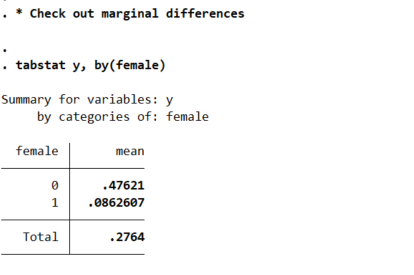So you can see males have the proportion of the outcome near 50% in the sample, whereas females are under 10%. So Calli is basically interested in the case below, where we estimate all pairwise interactions, so have many coefficient differences to test on the right hand side.

``````* Estimate model with interactions (linear coefficients)
logistic y i.female x1 x2 x3 i.female#(c.x1 c.x2 c.x3), coef``````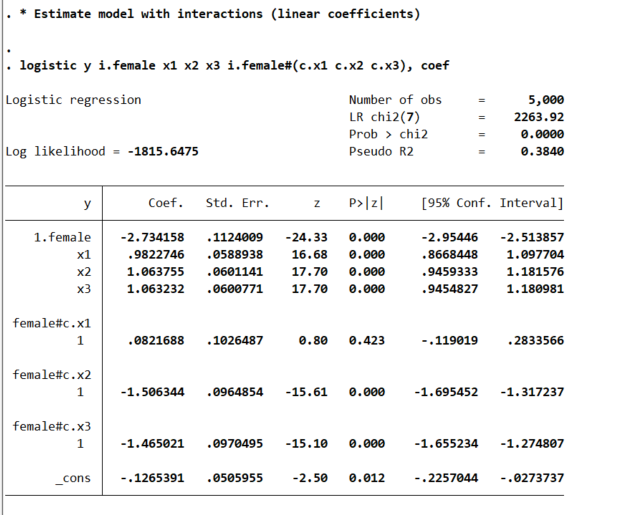This particular model does the Wald tests for the coefficient differences just by the way we have set up the model. So the interaction effects test for differences from the baseline model, so can see the interaction for x1 is not stat significant, but x2/x3 are (as they should be). But if you are interested in the marginal effects, one place to start is with derivatives directly, and Stata automatically for logit models spits out probabilities:

``````* Marginal effects
margins female, dydx(x1 x2 x3)
* x1 is the same linear effect, but margins are quite different``````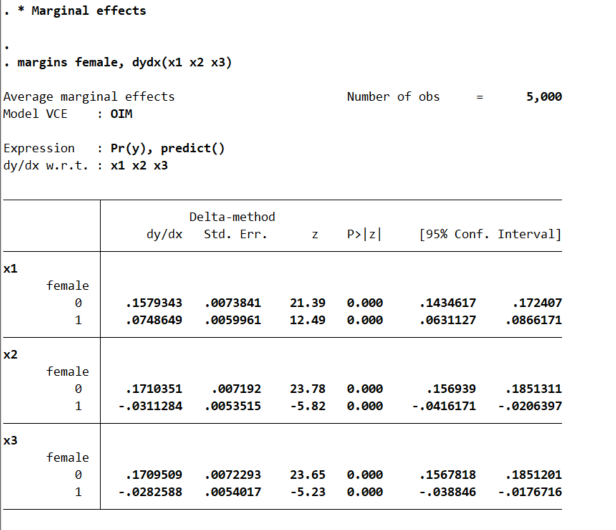So even though I made the underlying effect for x1 equal between males/females for the true underlying data generation process, you can see here the marginal derivative is much smaller for females. This is the main difference between Wald tests and margins.

This is ok though for many situations. Say x1 is a real valued treatment, such as Y is victimization in a sample of high risk youth, and x1 is hours given for a summer job. We want to know the returns of expanding the program – here the returns are higher for males than females due to the different baseline probabilities of risk between the two. This is true even if the relative effect of summer job hours is the same between the two groups.

Again Stata is very convenient, and we can test for the probability differences in males/females by appending `r.` to the front of the margins command.

``````* can test difference contrast in groups
margins r.female, dydx(x1 x2 x3)``````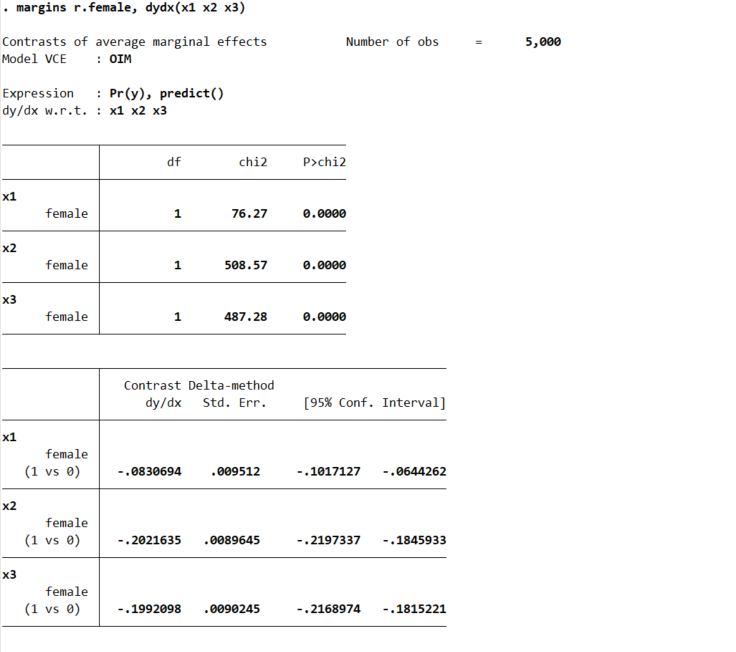But the marginal derivative can be difficult to interpret – it is the average slope, but what does that mean exactly? So I like evaluating at fixed points of the continuous variable. Going back to our summer job hours example, you could evaluate going from 0 to 50 hours, or going from 50 to 100, or 0 to 100, etc. and see the average returns in terms of reductions in the probability of trauma. Here because I simulated the data to be a standard normal, I just go from -1 to 0 to 1:

``````* Probably easier to understand at particular x1 values
margins female, at(x1=(-1(1)1))``````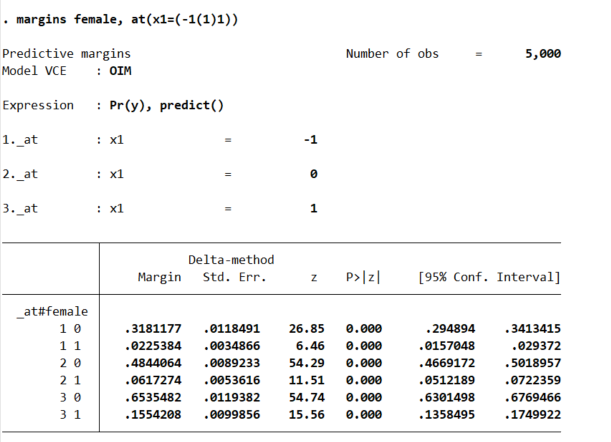So that table is dense, but it says when x1=-1, females have a probability of y of 2%, and males have a probability of y of 32%. Now go up the ladder to x1=0, females have a probability of 6% and males have a probability of 48%. So a discrete change of 4% for females and 16% for males. If we want to generate an interval around that discrete change effect:

``````* Can test increases one by one
margins female, at(x1=(-1 0 1))   contrast(atcontrast(ar) effects marginswithin)``````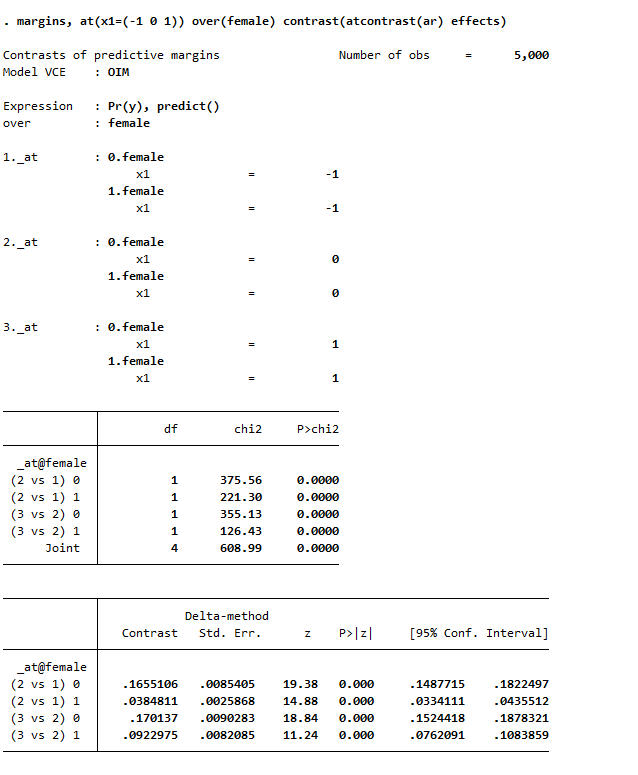See, isn’t Stata’s margins command so nice! (For above, it actually may make more sense to use `margins , at(x1=(-1 0 1)) over(female) contrast(atcontrast(ar) effects)`. Margins estimates the changes over the whole sample and averages filling in certain values, with over it only does the averaging within each group on over.) And finally we can test the difference in difference, to see if the discrete changes in males females from going from -1 to 0 to 1 are themselves significant:

``````* And can test increases of males vs females
margins r.female, at(x1=(-1(1)1)) contrast(atcontrast(ar))``````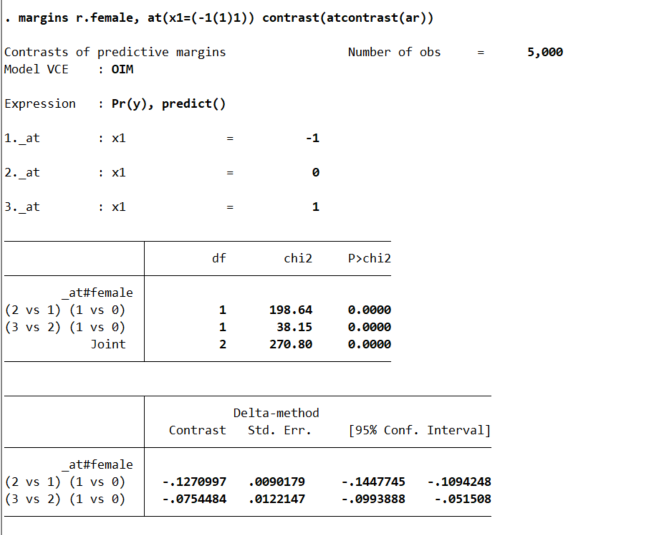So the increase in females is 13% points smaller than the increase in males going from -1 to 0, etc.

So I have spent alot of time on the probabilities so far. I find them much easier to interpret, and I do not care so much about the fact that it doesn’t necessarily say the underlying DGP is different from males/females. But many people are interested in the odds ratios (say in case-control studies). Or generalizing to a different sample, say this is a low risk sample of females, and you want to generalize the odds ratio’s to a higher risk sample with a baseline more around 50%. Then looking at the odds ratio may make more sense.

Or so far I have not even gotten to Calli’s main point, how to test many of these effects for no differences at once. There I would just suggest the likelihood ratio test (which does not have the problems with the Wald tests on the coefficients and that the variance estimates may be off):

``````* Estimate restricted model
logistic y ibn.female c.x1 c.x2 c.x3, coef noconstant
estimates store restrict

* Another way to do the full interaction model
* More like separate male and female
logistic y ibn.female ibn.female#(c.x1 c.x2 c.x3), coef noconstant
estimates store full

* LRT test between models
lrtest restrict full``````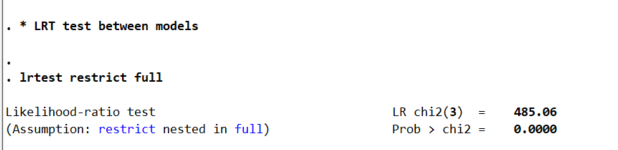So here as expected, one rejects the null that the restricted model is a better fit to the data. But this is pretty uninformative – I rather just go to the more general model and quantify the differences.

So if you really want to look at the odds ratios, we can do that using `lincom` post our full interaction model:

``logistic y i.female ibn.female#(c.x1 c.x2 c.x3), coef noconstant``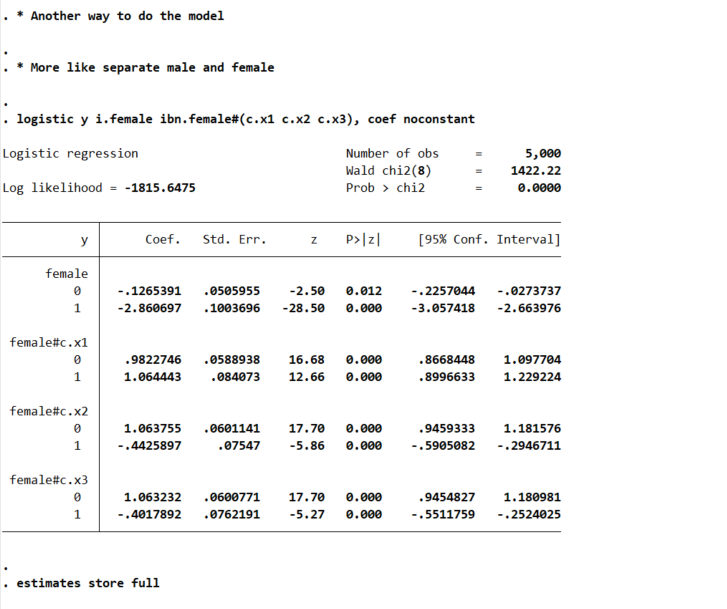And here is an example post Wald test for equality:

``lincom 0.female#c.x1 - 1.female#c.x1``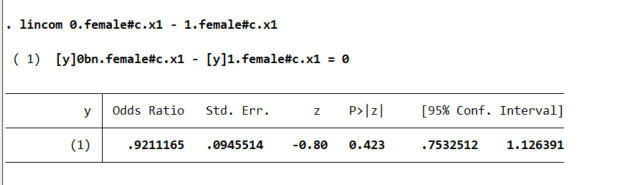You may ask where does this odd’s ratio of 0.921 come from? Well, way back in our first full model, the interaction term for `female*x1` is `0.0821688`, and `exp(-0.0821688)` equals that odds ratio and has the same p-value as the original model I showed. And so you can see that the x1 effect is the same across each group. But estimating the other contrasts is not:

``lincom 0.female#c.x2 - 1.female#c.x2``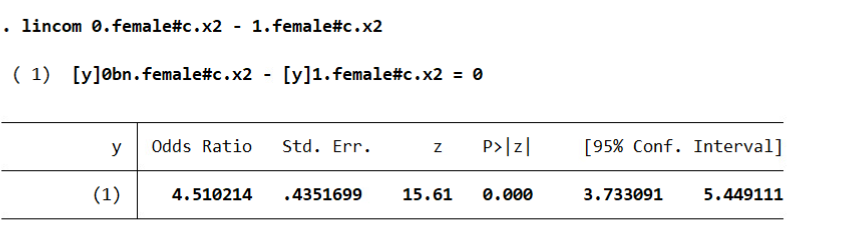And Stata defaults this to estimating a difference in the odds ratio (as far as I can tell you can’t tell Stata to do the linear coefficient after logit, would need to change the model to `glm y x, family(binomial) link(logit)` to do the linear tests).

I honestly don’t know how to really interpret this – but I have been asked for it several different times by clients. Maybe they know better than me, but I think it is more to do with people just expect to be dealing with odds ratios after a logistic regression.

So we can coerce margins to give us odds ratios:

``````* For the odds ratios
quietly margins female, at(x3=(-1(0.1)1)) expression(exp(predict(xb)))
marginsplot , yscale(log) ylabel(0.125 0.25 0.5 1 2 4 8)``````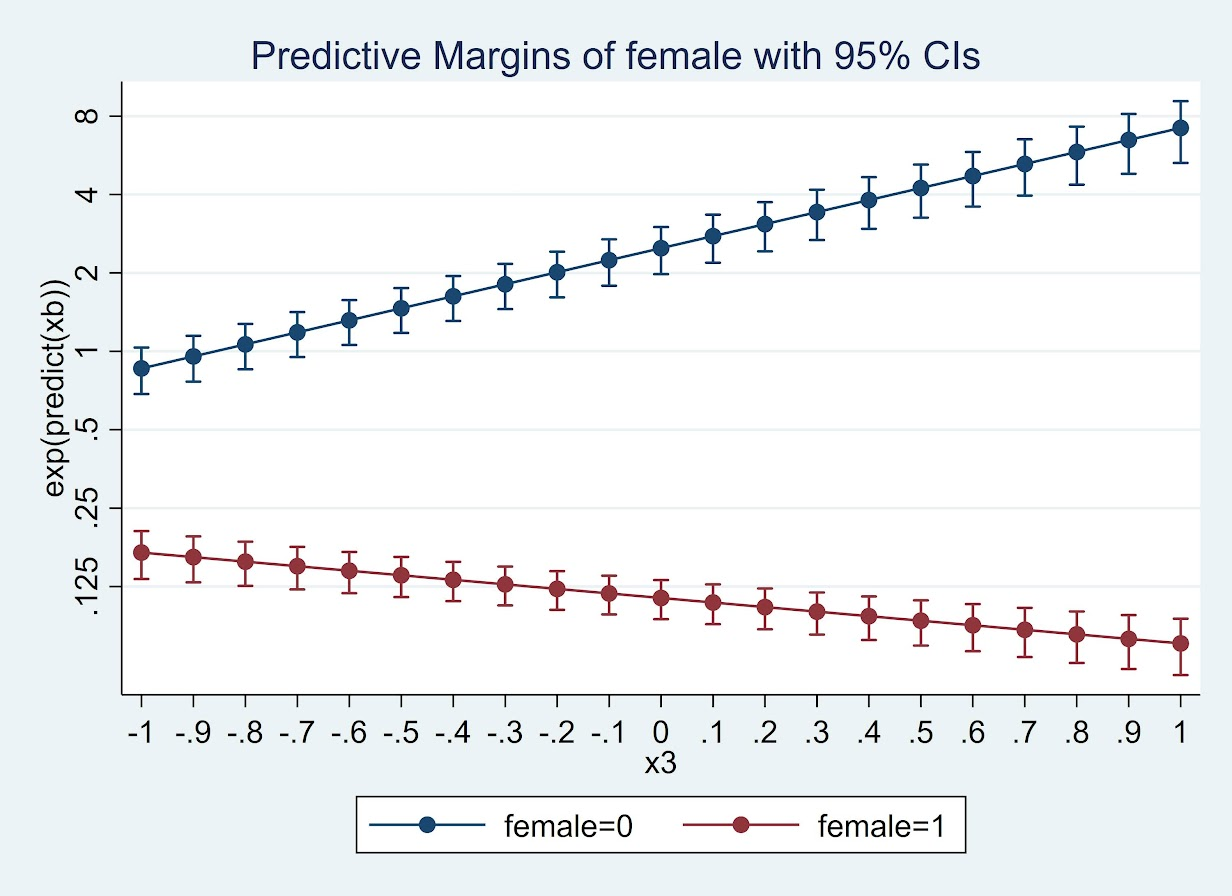Or give us the differences in the odds ratio:

``````* For the contrast in the OR
quietly margins r.female, at(x3=(-1(0.1)1)) expression(exp(predict(xb)))
marginsplot``````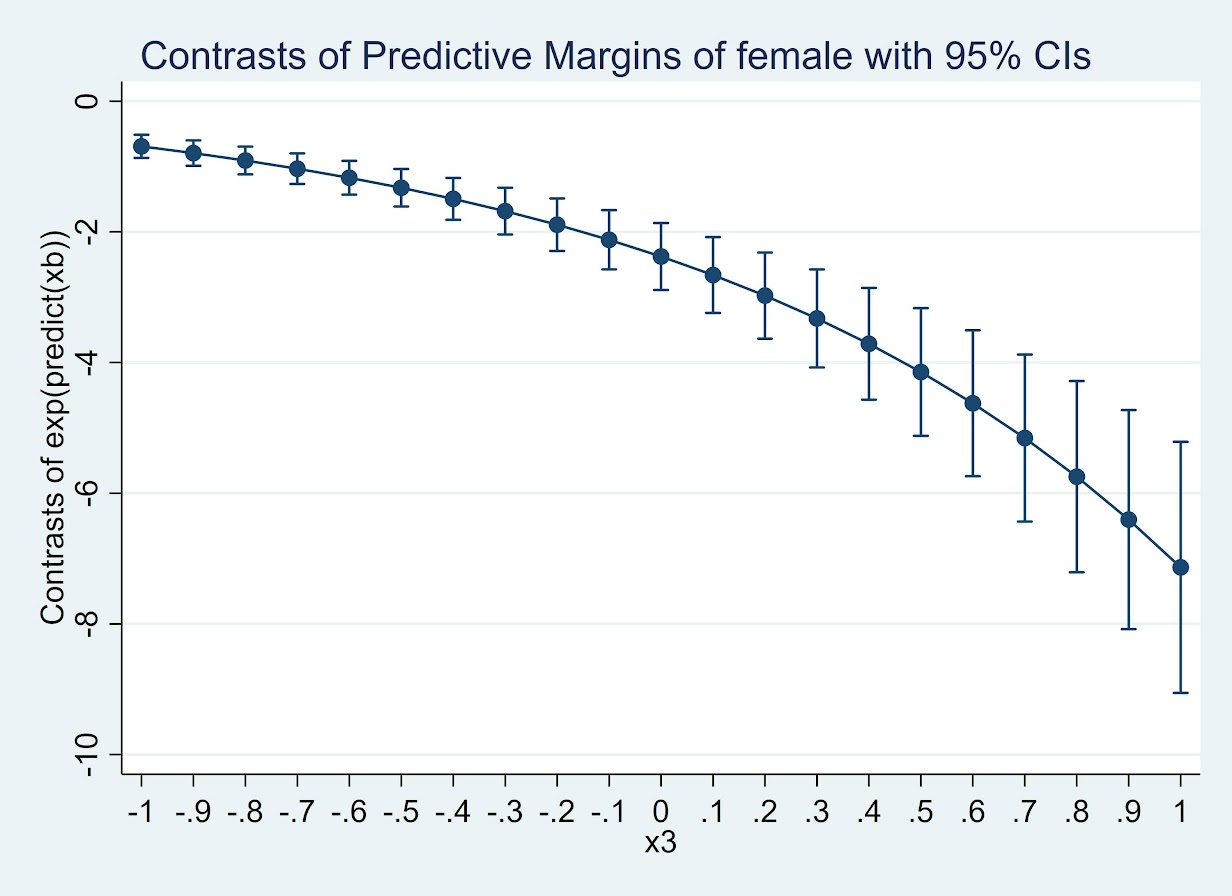(Since it is a negative number cannot be drawn on a log scale.) But again I find it much easier to wrap my head around probabilities:

``````* For the probabilities
quietly margins female, at(x3=(-2(0.1)2))
marginsplot``````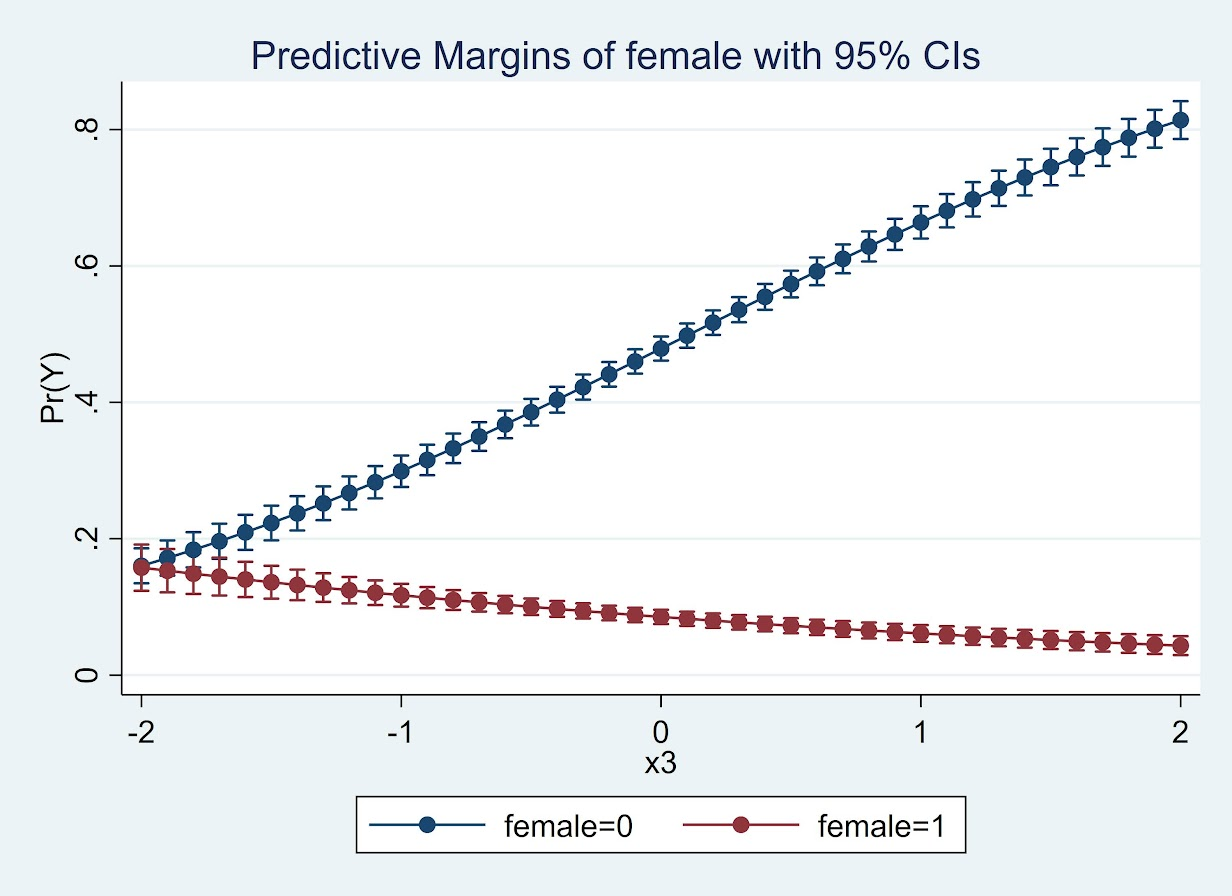So here while x3 increases, for males it increases the probability and females it decreases. The female decrease is smaller due to the smaller baseline risk in females.

So while Calli’s original question was how to do this reasonably for many different contrasts, I would prefer the actual empirical estimates of the differences. Doing a single contrast among a small number of representative values over many variables and placing in a table/graph I think is the best way to reduce the complexity.

I just don’t find the likelihood ratio tests for all equalities that informative, and for large samples they will almost always say the more flexible model is better than the restricted model.

We estimate models to actually look at the quantitative values of those estimates, not to do rote hypothesis testing.

# Some microsynth notes

My question is from our CPP project on business improvement districts (Piza, Wheeler, Connealy, Feng 2020). The article indicates that you ran three of the microsynth matching variables as an average over each instead of the cumulative sum (street length, percent new housing structures, percent occupied structures). How did you get R to read the variables as averages instead of the entire sum of the treatment period of interest? I have the microsynth code you used to generate our models, but cannot seem to determine how you got R to read the variables as averages.

So Nate is talking about this paper, Crime control effects of a police substation within a business improvement district: A quasi-experimental synthetic control evaluation (Piza et al., 2020), and here is the balance table in the paper: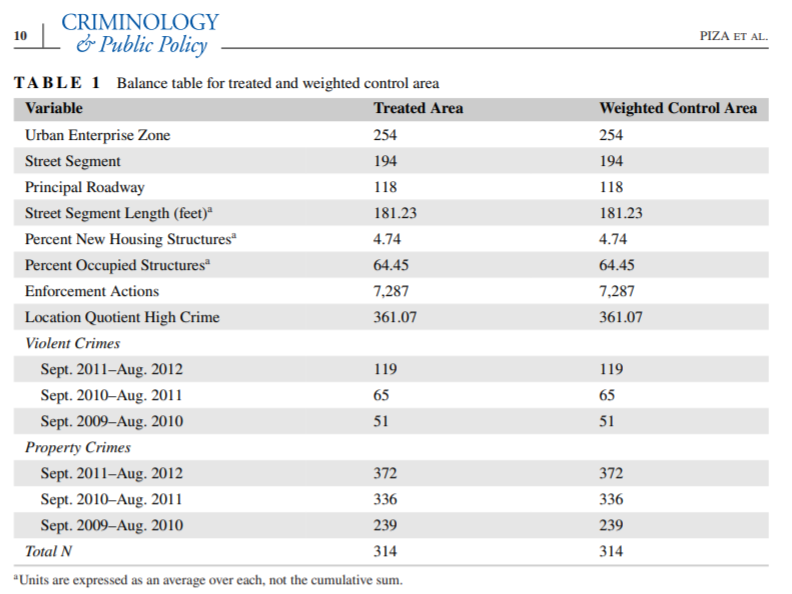To be clear to folks, I did not balance on the averages, but simply reported the table in terms of averages. So here is the original readout from R: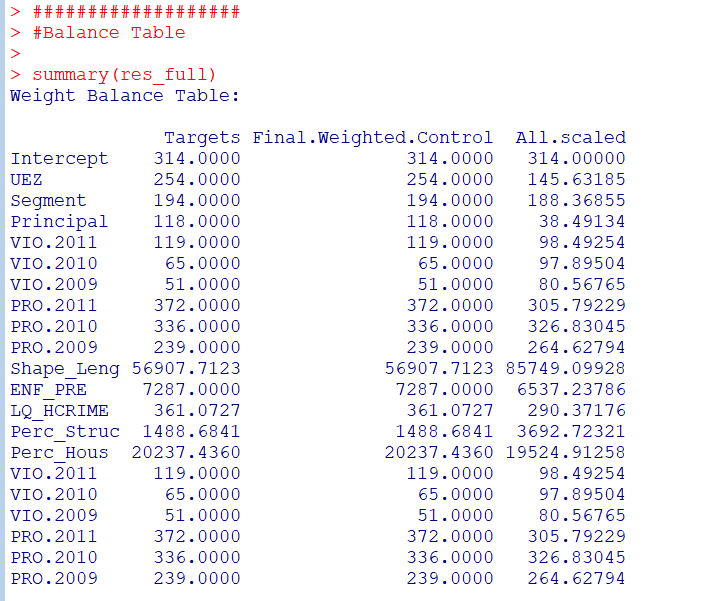So I just divided those noted rows by 314 to make them easier to read. You could divide values by the total number of treated units though in the original data to have microsynth match on the averages instead if you wanted to. Example below (this is R code, see the microsynth library and paper by Robbins et al., 2017):

``````library(microsynth)
set.seed(10)

data(seattledmi) #just using data in the package
cs <- seattledmi
# calculating proportions
cs\$BlackPerc <- (cs\$BLACK/cs\$TotalPop)*100
cs\$FHHPerc <- (cs\$FEMALE_HOU/cs\$HOUSEHOLDS)*100
# replacing 0 pop with 0
cs[is.na(cs)] <- 0

cov.var <- c("TotalPop","HISPANIC","Males_1521","FHHPerc","BlackPerc")
match.out <- c("i_felony", "i_misdemea")

sea_prop <- microsynth(cs,
idvar="ID", timevar="time", intvar="Intervention",
start.pre=1, end.pre=12, end.post=16,
match.out.min=match.out,match.out=FALSE,
match.covar=FALSE,check.feas=FALSE,
match.covar.min=cov.var,
result.var=match.out)

summary(sea_prop) # balance table``````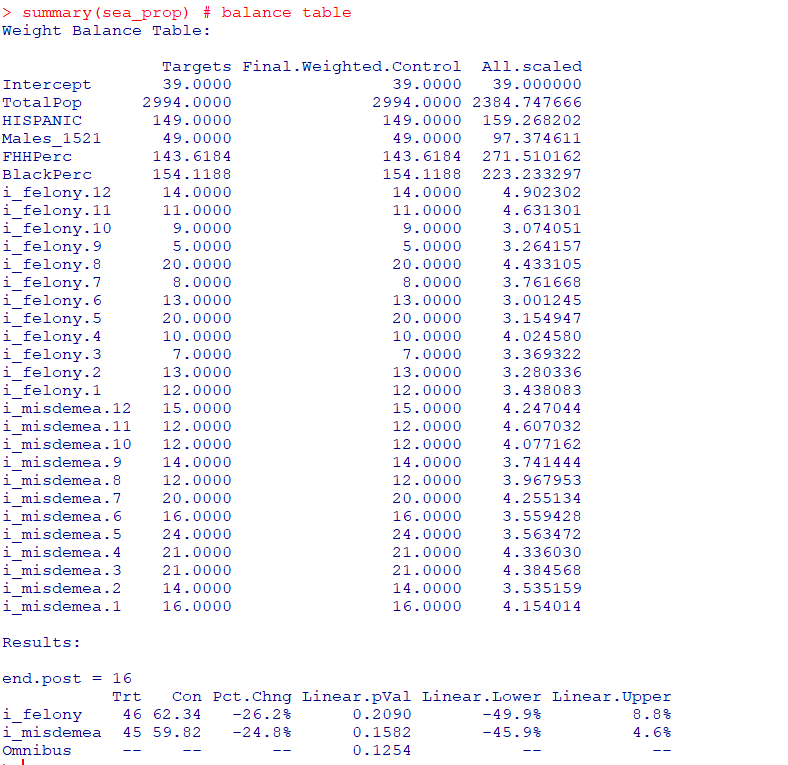And here you can see that we are matching on the cumulative sums for each of the areas, but we can divide our covariates by the number of treated units, and we will match on the proportional values.

``````# Can divide by 39 and get the same results
cs[,cov.var] <- cs[,cov.var]/39

sea_div <- microsynth(cs,
idvar="ID", timevar="time", intvar="Intervention",
start.pre=1, end.pre=12, end.post=16,
match.out.min=match.out,match.out=FALSE,
match.covar=FALSE,check.feas=FALSE,
match.covar.min=cov.var,
result.var=match.out)

summary(sea_div) # balance table``````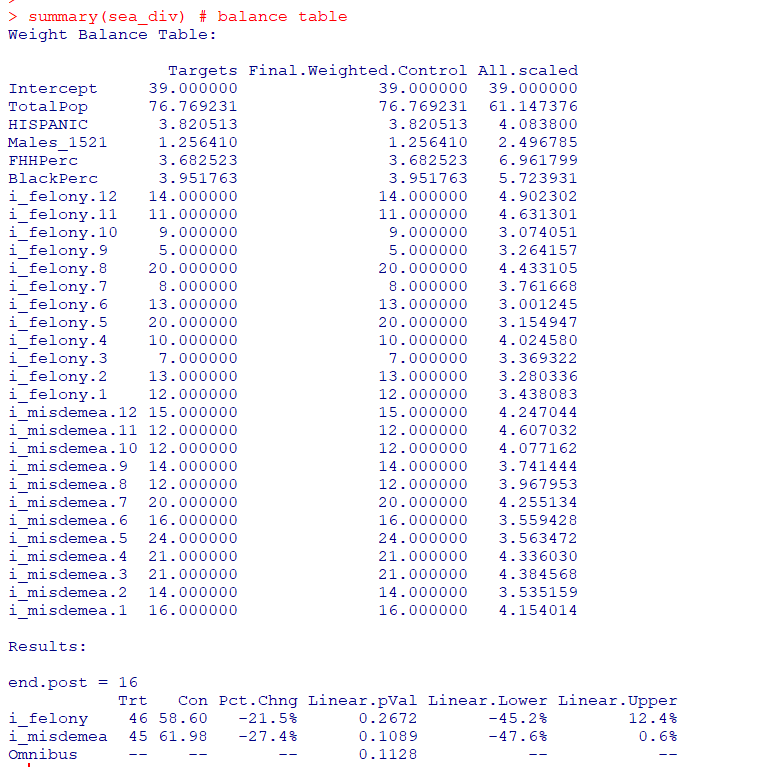Note that these do not result in the same weights. If you look at the results you will see the treatment effects are slightly different. Also if you do:

``````# Showing weights are not equal
all.equal(sea_div\$w\$Weights,sea_prop\$w\$Weights)``````

It does not return True. Honestly not familiar enough with the procedure that microsynth uses to do the matching (Raking survey weights) to know if this is due to stochastic stuff or due to how the weighting algorithm works (I would have thought a linear change does not make a difference, but I was wrong).

On the bucket list is to do a matching algorithm that returns geographically contiguous areas and gives the weights all values of 1 (so creates comparable neighborhoods), instead of estimating Raking weights. That may be 5 years though before I get around to that. Gio has a nice map to show the way the weights work now is they may be all over the place (Circo et al., 2021) – I am not sure that is a good thing though.

But I did want to share some functions I used for the paper I worked with Nate on. First, this is for if you use the permutation approach, the function `prep_synth` returns some of the data in a nicer format to make graphs and calculate your own stats:

``````# Function to scoop up the data nicely
prep_synth <- function(mod){
#Grab the plot data
plotStats <- mod[['Plot.Stats']]
#For the left graph
Treat <- as.data.frame(t(plotStats\$Treatment))
Treat\$Type <- "Treat"
#This works for my data at years, will not
#Be right for data with more granular time though
Treat\$Year <- as.integer(rownames(Treat))
Cont <- as.data.frame(t(plotStats\$Control))
Cont\$Type <- "Control"
Cont\$Year <- as.integer(rownames(Cont))
AllRes <- rbind(Treat,Cont)
#For the right graph
Perm <- as.data.frame(t(as.data.frame(plotStats\$Difference)))
SplitStr <- t(as.data.frame(strsplit(rownames(Perm),"[.]")))
colnames(SplitStr) <- c("Type","Year")
rownames(SplitStr) <- 1:nrow(SplitStr)
SplitStr <- as.data.frame(SplitStr)
Perm\$Type <- as.character(SplitStr\$Type)
Perm\$Year <- as.integer(as.character(SplitStr\$Year))
Perm\$Group <- ifelse(Perm\$Type == 'Main','Treatment Effect','Permutations')
#Reordering factor levels for plots
AllRes\$Type <- factor(AllRes\$Type,levels=c('Treat','Control'))
levels(AllRes\$Type) <- c('Treated','Synthetic Control')
Perm\$Group <- factor(Perm\$Group,levels=c('Treatment Effect','Permutations'))
#Exporting result
Res <- vector("list",length=2)
Res[] <- AllRes
Res[] <- Perm
names(Res) <- c("AggOutcomes","DiffPerms")
return(Res)
}``````

It works for the prior tables, but I really made these functions to work with when you used permutations to get the errors. (In the micro synth example, it is easier to work with permutations than in the state level example for synth, in which I think conformal prediction intervals makes more sense, see De Biasi & Circo, 2021 for a recent real example with micro place based data though.)

``````# Takes like 1.5 minutes
sea_perm <- microsynth(seattledmi,
idvar="ID", timevar="time", intvar="Intervention",
start.pre=1, end.pre=12, end.post=16,
match.out.min=match.out,match.out=FALSE,
match.covar=FALSE,check.feas=FALSE,
match.covar.min=cov.var,
result.var=match.out, perm=99)

res_prop <- prep_synth(sea_perm)
print(res_prop)``````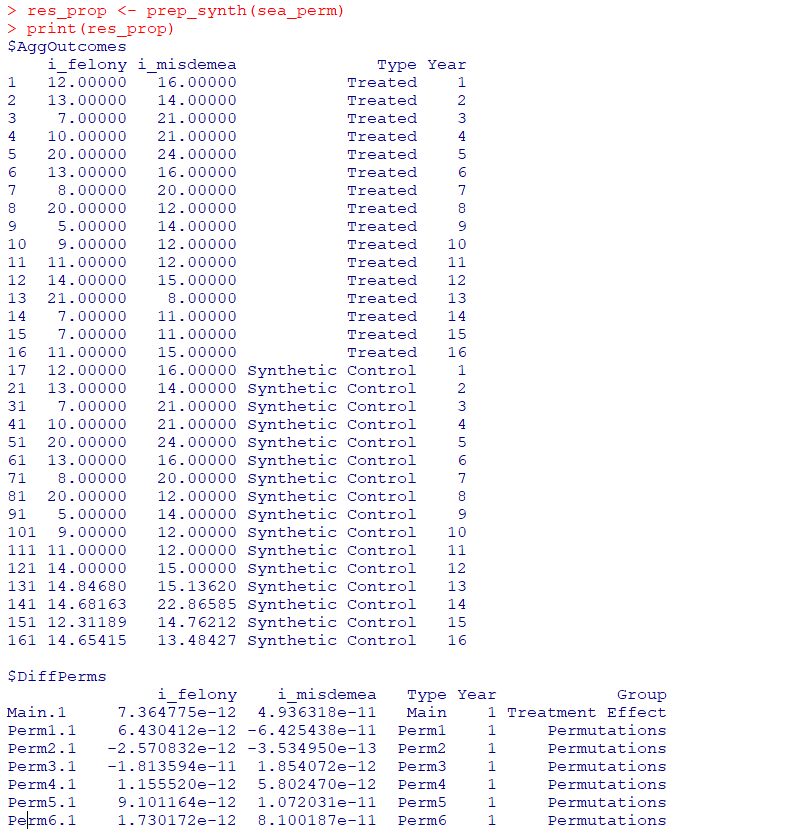So the dataframe in the first slot is the overall treatment effect, and the second dataframe is a nice stacked version for the permutations. First, I really do not like the percentage change (see Wheeler, 2016 for the most direct critique, but I have a bunch on this site). So I wrote code to translate the treatment effects into crime count reductions instead of the percent change stuff.

``````# Getting the observed treatment effect on count scale
# vs the permutations

agg_fun <- function(x){
sdx <- sd(x)
minval <- min(x)
l_025 <- quantile(x, probs=0.025)
u_975 <- quantile(x, probs=0.975)
maxval <- max(x)
totn <- length(x)
res <- c(sdx,minval,l_025,u_975,maxval,totn)
return(res)
}

treat_count <- function(rp){
# Calculating the treatment effect based on permutations
keep_vars <- !( names(rp[]) %in% c("Year","Group") )
out_names <- names(rp[])[keep_vars][1:(sum(keep_vars)-1)]
loc_dat <- rp[][,keep_vars]
agg_treat <- aggregate(. ~ Type, data = loc_dat, FUN=sum)
n_cols <- 2:dim(agg_treat)
n_rows <- 2:nrow(agg_treat)
dif <- agg_treat[rep(1,max(n_rows)-1),n_cols] - agg_treat[n_rows,n_cols]
dif\$Const <- 1
stats <- aggregate(. ~ Const, data = dif, FUN=agg_fun)
v_names <- c("se","min","low025","up975","max","totperm")
long_stats <- reshape(stats,direction='long',idvar = "Const",
varying=list(2:ncol(stats)),
v.names=v_names, times=out_names)
# Add back in the original stats
long_stats <- long_stats[,v_names]
rownames(long_stats) <- 1:nrow(long_stats)
long_stats\$observed <- t(agg_treat[1,n_cols])[,1]
long_stats\$outcome <- out_names
ord_vars <- c('outcome','observed',v_names)
return(long_stats[,ord_vars])
}

treat_count(res_prop)``````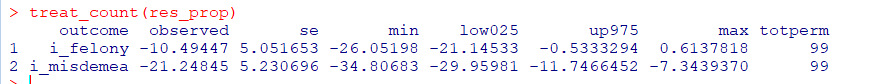So that is the cumulative total effect of the intervention. This is more similar to the WDD test (Wheeler & Ratcliffe, 2018), but since the pre-time period is matched perfectly, just is the differences in the post time periods. And here it uses the permutations to estimate the error, not any Poisson approximation.

But I often see folks concerned about the effects further out in time for synthetic control studies. So here is a graph that just looks at the instant effects for each time period, showing the difference via the permutation lines:

``````# GGPLOT graphs, individual lines
library(ggplot2)
perm_data <- res_prop[]
# Ordering factors to get the treated line on top
perm_data\$Group <- factor(perm_data\$Group, c("Permutations","Treatment Effect"))
perm_data\$Type <- factor(perm_data\$Type, rev(unique(perm_data\$Type)))
pro_perm <- ggplot(data=perm_data,aes(x=Year,y=i_felony,group=Type,color=Group,size=Group)) +
geom_line() +
scale_color_manual(values=c('grey','red')) + scale_size_manual(values=c(0.5,2)) +
geom_vline(xintercept=12) + theme_bw() +
labs(x=NULL,y='Felony Difference from Control') +
scale_x_continuous(minor_breaks=NULL, breaks=1:16) +
scale_y_continuous(breaks=seq(-10,10,2), minor_breaks=NULL) +
theme(panel.grid.major = element_line(linetype="dashed"), legend.title= element_blank(),
legend.position = c(0.2,0.8), legend.background = element_rect(linetype="solid", color="black")) +
theme(text = element_text(size=16), axis.title.y=element_text(margin=margin(0,10,0,0)))``````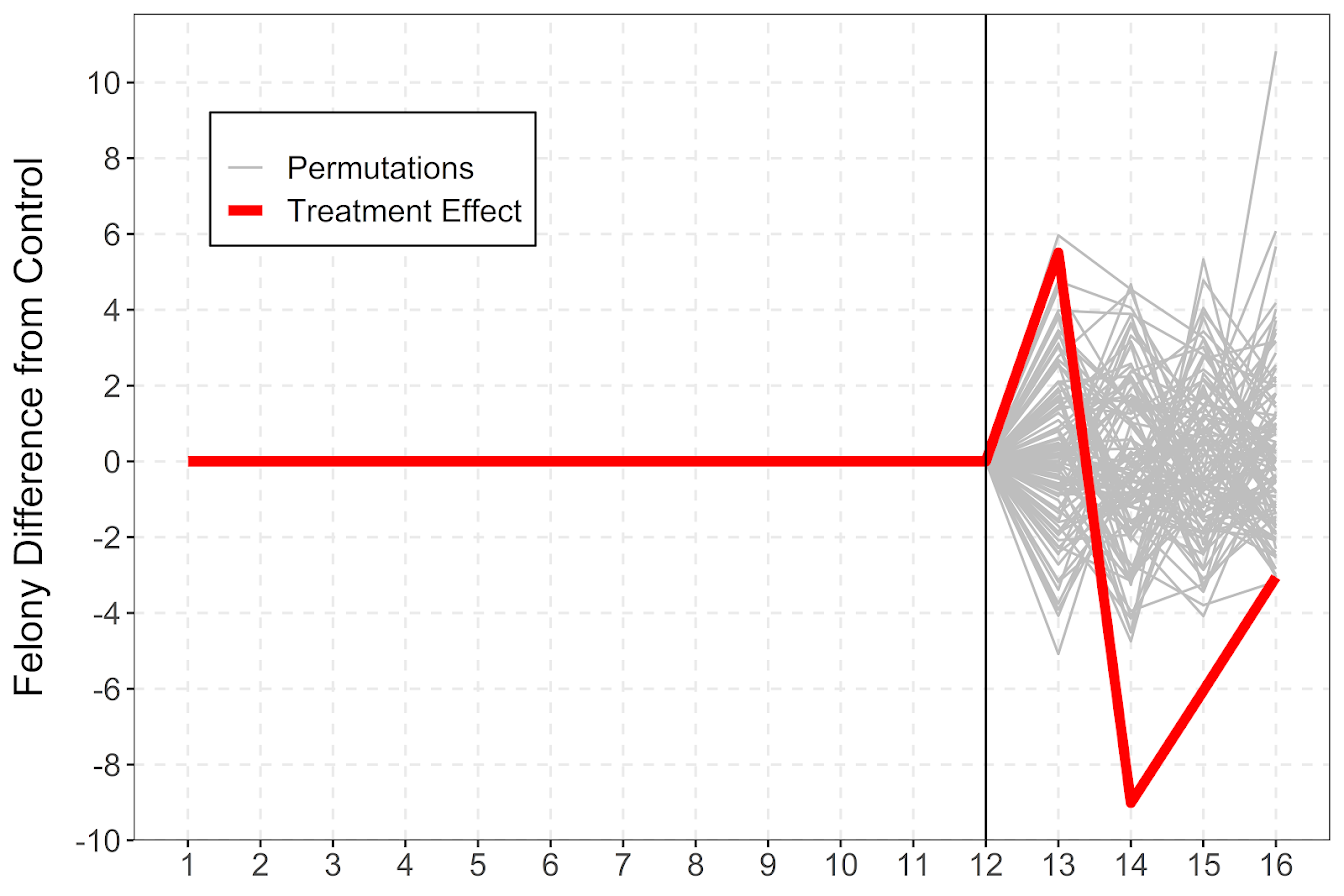And I also like looking at this for the cumulative effects as well, which you can see with the permutation lines widen over time.

``````# Cumulative vs Pointwise
perm_data\$csum_felony <- ave(perm_data\$i_felony, perm_data\$Type, FUN=cumsum)
pro_cum  <- ggplot(data=perm_data,aes(x=Year,y=csum_felony,group=Type,color=Group,size=Group)) +
geom_line() +
scale_color_manual(values=c('grey','red')) + scale_size_manual(values=c(0.5,2)) +
geom_vline(xintercept=12) + theme_bw() +
labs(x=NULL,y='Felony Difference from Control Cumulative') +
scale_x_continuous(minor_breaks=NULL, breaks=1:16) +
scale_y_continuous(breaks=seq(-20,20,5), minor_breaks=NULL) +
theme(panel.grid.major = element_line(linetype="dashed"), legend.title= element_blank(),
legend.position = c(0.2,0.8), legend.background = element_rect(linetype="solid", color="black")) +
theme(text = element_text(size=16), axis.title.y=element_text(margin=margin(0,10,0,0)))``````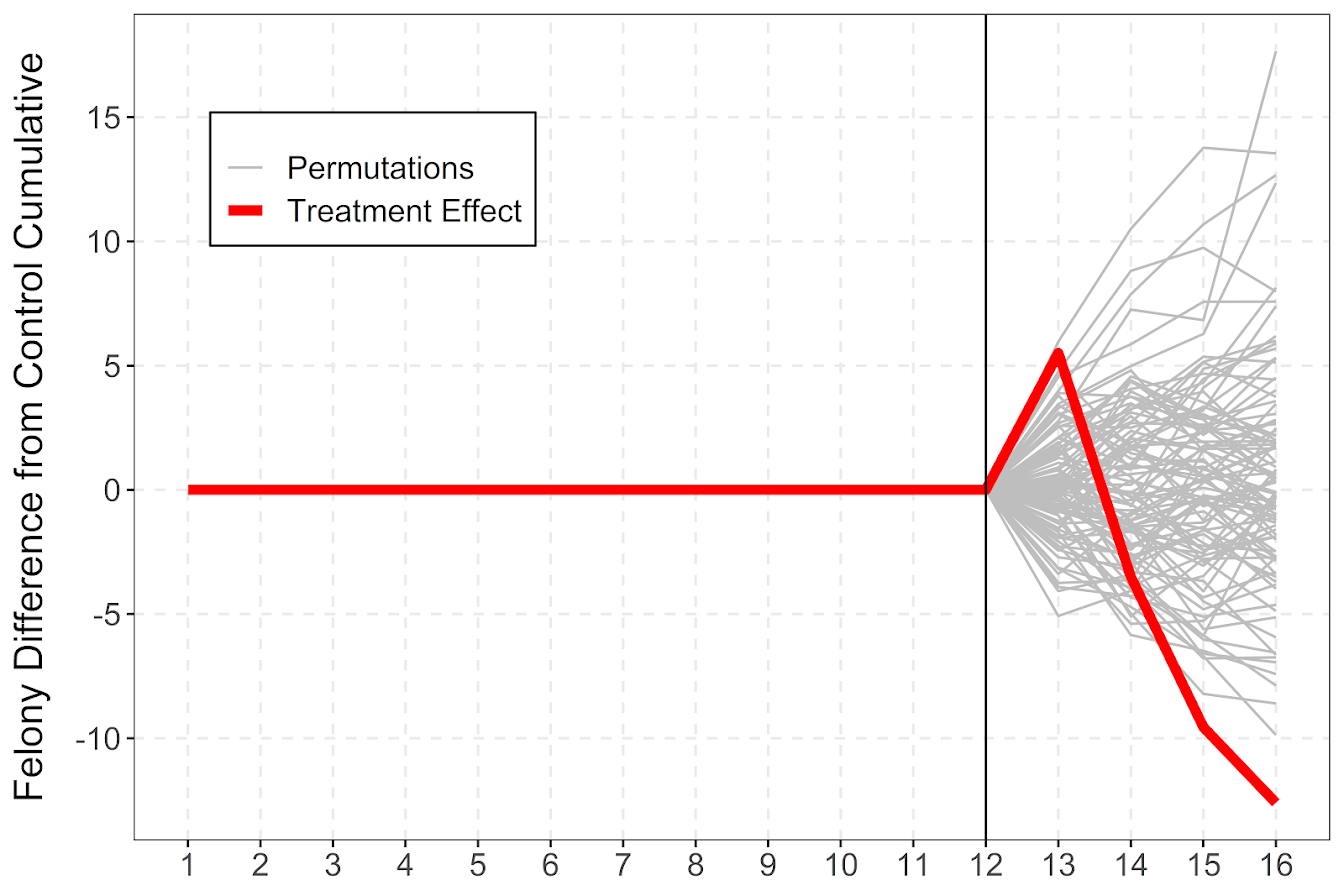If you do a ton of permutations (say 999 instead of 99), it would likely make more sense to do a fan chart type error bars and show areas of different percentiles instead of each individual line (Yim et al., 2020).

I will need to slate a totally different blog post to discuss instant vs cumulative effects for time series analysis. Been peer-reviewing quite a few time series analyses of Covid and crime changes – most everyone only focuses on instant changes, and does not calculate cumulative changes. See for example estimating excess deaths for the Texas winter storm power outage (Aldhous et al., 2021). Folks could do similar analyses for short term crime interventions. Jerry has a good example of using the Causal Impact package to estimate cumulative effects for a gang takedown intervention (Ratcliffe et al., 2017) for one criminal justice example I am familiar with.

Again for folks feel free to ask me anything. I may not always be able to do as deep a dive as this, but always feel free to reach out.

# Using google places API in criminology research?

In my ask me anything series, Thom Snaphaan, a criminologist at Ghent University writes in with this question (slightly edited by me):

I read your blog post on using the Google Places API for criminological research. I am interested in using these data in the context of my PhD research. Can I ask you some questions on this matter? We think Google Places might be a very rich data source, specifically the user ratings of places. (1) Is it allowed to use these data on a large scale (two large cities) for scientific research? (2) Is it possible to download a set without the limit of 1,000 requests per day? (3) Are there, in your experience, other (perhaps more interesting) data sources to conduct this study? Many thanks! Best, Thom

And for my responses to Thom,

For 1) I believe it is OK to use for research purposes. You are not allowed to download the data and resell it though.

For 2) The quotas for the places API are much larger, it is now you get \$200 credit per month, which amounts to 100,000 API calls. So that should be sufficient even for a large city.

For 3) I do not know, I haven’t paid much attention to the different online apps that do user reviews. Here in the states we have another service called Yelp (mostly for restaurants), I am not sure if that has more reviews or not though.

One additional piece of information not commonly used in place based research (but have seen it used some Hipp, 2016; Perenzin-Askey, 2018), is the use of the number of employees or sales volume at particular crime generators/attractors. This is not available via google, but is via Reference USA or Lexis Nexis. For Dallas IIRC Reference USA had much better coverage (almost twice as many businesses), but I recently reviewed a paper that did boots on the ground validation for Google data in the Indian city of Chennai and the validation for google businesses was very high (Kuralarason & Bernasco, 2021)

Answer in the comments if you think you have more helpful information on leveraging the place based user reviews in research projects.

In the past I have written about using various google APIs, and which I have used in my research for several different projects.

Google has new pricing now, where you get \$200 in credits per month per API. But overall the Places and the streetview API you get a crazy ton of potential calls, so will work for most research projects. Looking it over I actually don’t think I have used Google places data in any projects, in Wheeler & Steenbeek, 2021 I use reference USA and some other sources.

Geocoding and distance API limits are tougher, I ended up accidentally charging myself ~\$150 for my work with Gio on gunshot fatalities (Circo & Wheeler, 2021) calculating network distance and approximate drive times. The vision API is also quite low (1000 per month), so will need to budget/plan if you need those services for your project. Geocoding you should be able to find alternatives, like the census geocoder (R, python) and then only use google for the leftovers.

# References

• Circo, G. M., & Wheeler, A. P. (2021). Trauma Center Drive Time Distances and Fatal Outcomes among Gunshot Wound Victims. Applied Spatial Analysis and Policy, 14(2), 379-393.
• Hipp, J. R. (2016). General theory of spatial crime patterns. Criminology, 54(4), 653-679.
• Kuralarasan, K., & Bernasco, W. (2021). Location Choice of Snatching Offenders in Chennai City. Journal of Quantitative Criminology, Online First.
• Perezin-Askey, A., Taylor, R., Groff, E., & Fingerhut, A. (2018). Fast food restaurants and convenience stores: Using sales volume to explain crime patterns in Seattle. Crime & Delinquency, 64(14), 1836-1857.
• Wheeler, A. P., & Steenbeek, W. (2021). Mapping the risk terrain for crime using machine learning. Journal of Quantitative Criminology, 37(2), 445-480.